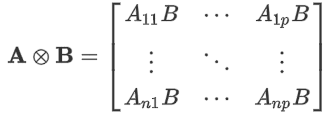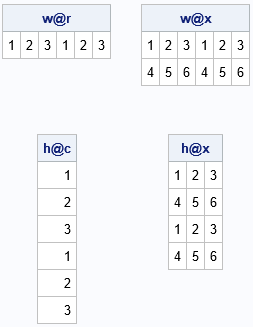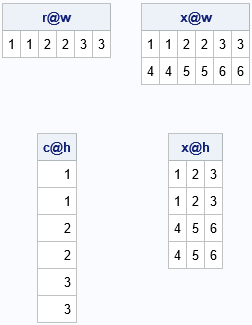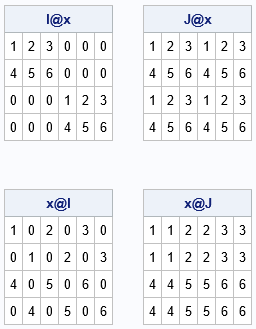The Kronecker product (also called the direct product) is a binary operation that combines two matrices to form a new matrix. The Kronecker product appears in textbooks about the design of experiments and multivariate statistics. The Kronecker product seems intimidating at first, but often one of the matrices in the product has a special form, such as being a matrix of all 1s. In these special cases, the Kronecker product becomes much easier to understand. This article demonstrates some of the most common uses of the Kronecker product, including using it to concatenate matrices and to form block matrices.

### What is the Kronecker product?

The Kronecker product is best understood by thinking about block matrices. If A is a n x p matrix and B is a m x q matrix, then the Kronecker product A ⊗ B is a block matrix that is formed from blocks of B, where each block is multiplied by an element of A:The SAS/IML language uses the "at sign" (@) to represent the binary operator. Thus, the SAS/IML expression A @ B forms the Kronecker product A ⊗ B.

### The Kronecker product for vectors of 1s and special matrices

If A or B has a special form, the Kronecker product simplifies. First, consider only the case where A is a vector of all 1s or a special matrix. The following statements follow directly from the definition of the Kronecker product.

• If A is a row vector of all 1s, then A ⊗ B is the horizontal concatenation of p copies of B.
• If A is a column vector of all 1s, then A ⊗ B is the vertical concatenation of n copies of B.
• If A is a matrix of all 1s, then A ⊗ B is a block matrix that contains blocks of the matrix B.
• If A is the identity matrix, then A ⊗ B is a block diagonal matrix that contains copies of the matrix B along the diagonal.

Next, let A be an arbitrary matrix but consider only special forms for B:

• If B is a row vector of all 1s, then A ⊗ B contains q copies of the first column of A, followed by q copies of the second column of A, and so forth. I call this sequential replication of columns of A.
• If B is a column vector of all 1s, then A ⊗ B contains m copies of the first row of A, followed by m copies of the second row of A, and so forth. This is sequential replication of rows of A.
• If B is a matrix of all 1s, then A ⊗ B is a block matrix where the (i,j)th block is the constant matrix where all elements are A[i,j].
• If B is an identity matrix, then A ⊗ B is a block matrix where the (i,j)th block is the matrix A[i,j]*I.

The next sections show concrete examples of these eight ways to use the Kronecker product operator.

### Use the Kronecker product for horizonal or vertical concatenation

You can use the Kronecker product to perform horizontal or vertical concatenation. For example, the following SAS/IML program defines two vectors that contain only 1s. The vector w is a row vector and the vector h is a column vector. The program computes w ⊗ B and h ⊗ B for various choices of B. The ODS LAYOUT GRIDDED statement arranges the output in a grid.

```proc iml; /* specify RHS vectors */ w = {1 1}; /* w for 'wide' (row vector) */ h = {1, 1}; /* h for 'high' (col vector) */   /* specify data matrices */ r = {1 2 3}; /* row vector */ c = {1, 2, 3}; /* column vector */ x = {1 2 3, /* 2 x 3 matrix */ 4 5 6};   /******************************/ /* vector @ B : Concatenation */ /******************************/ wr = w @ r; /* horiz concat. Same as repeat(r, 1, ncol(w)) */ wx = w @ x; /* horiz concat. Same as repeat(x, 1, ncol(w)) */ hc = h @ c; /* vert concat. Same as repeat(c, nrow(h), 1) */ hx = h @ x; /* vert concat. Same as repeat(x, nrow(h), 1) */   ods layout gridded columns=2 advance=table; print wr[L='w@r'], wx[L='w@x'], hc[L='h@c'], hx[L='h@x']; ods layout end;```The output indicates that the Kronecker product with w on the left results in horizontal concatenation. Using h on the left results in vertical concatenation.

### Use the Kronecker product for sequential replication

The previous section showed how to take a sequence of numbers such as {1,2,3} and repeat the entire sequence to form a new sequence such as {1,2,3,1,2,3}. But sometimes you want to repeat each element before repeating the next element. In other words, you might be interested in forming a sequence such as {1,1,2,2,3,3}. You can perform this kind of "sequential replication" by putting the data on the left and the vectors of 1s on the right, as follows:

```/***************************************/ /* A @ vector : Sequential replication */ /***************************************/ rw = r @ w; /* repeat cols sequentially */ xw = x @ w; ch = c @ h; /* repeat rows sequentially */ xh = x @ h;   ods layout gridded columns=2 advance=table; print rw[L='r@w'], xw[L='x@w'], ch[L='c@h'], xh[L='x@h']; ods layout end;```It is worth mentioning that the second row of output is the transpose of the first row. That is because the transpose operation "distributes" over the Kronecker product operator. That is, for any matrices A and B, it is true that (A ⊗ B)` = A` ⊗ B`. Since r`=c and w`=h, then (r ⊗ w)` = (r` ⊗ w`) = c ⊗ h.

### Use the Kronecker product to construct block matrices

The Kronecker product is essentially an operation that forms block matrices. When one of the components is a vector of all 1s, then "forming a block matrix" is the same as concatenation. But if A is a binary matrix, then A ⊗ B is a block matrix that has the same structure as A, but each nonzero block is a copy of B.

Probably the most important subcase is that the matrix I(n) ⊗ B is a block diagonal matrix, where each diagonal block is a copy of B. The following list gives some other examples that use the identity or a constant matrix. Let I(n) be the n x k identity matrix and let J(n) be the n x n matrix of all 1s. Then

• I(n) ⊗ B is a block diagonal matrix with copies of B on the diagonal.
• J(n) ⊗ B is a block matrix with a copy of B in each block.
• A ⊗ I(n) is a block matrix where each block is a diagonal matrix.
• A ⊗ J(n) is a block matrix where each block is a constant matrix.
```Ix = I(2) @ x; /* diagonal block matrix */ Jx = J(2) @ x; /* 2 x 2 block matrix */ xI = x @ I(2); /* blocks of diagonal matrices */ xJ = x @ J(2); /* blocks of constant matrices */   ods layout gridded columns=2 advance=table; print Ix[L='I@x'], Jx[L='J@x'], xI[L='x@I'], xJ[L='x@J']; ods layout end;```### How is the Kronecker product used in statistics?

Recall that (mathematically) you can only add matrices that have the same dimensions. For example, you can't add a matrix and a vector. However, a common operation in multivariate statistics is to center a data matrix by subtracting the mean of each column from that column. If X is the data matrix, "mean(X)" is a row vector where the i_th element of the vector is the mean of the i_th column. The centering operation is sometimes informally written as "X – mean(X)," even though that expression does not make mathematical sense. Formally, you need to vertically concatenate the mean vector n times, where n is the number of rows in the data matrix. As we have seen, if h is a column vector of length n that contains all 1s, then mean(X) ⊗ h is a matrix and the expression X – mean(X) ⊗ h is a proper mathematical expression that indicates the centering operation.

As described in a previous article, in the early days of SAS, statistical programmers had to use Kronecker product operator to ensure that all matrix operations were mathematically correct. But that changed in SAS version 8 (circa 1999) when the IML language introduced a shorthand notation that makes it easier to perform row and column operations. Back in the "old days," you saw lots of programs that used the Kronecker operator like this:

```/* read data matrix into X */ use Sashelp.Class; read all var _num_ into X; close; mean = x[:,]; /* row vector of means for each column */ h = j(nrow(X), 1, 1); /* column vector */ CenterX = X - mean @ h; /* subtract matrices of same dimension */```

The modern SAS/IML programmer will typically use the shorthand notation to avoid the Kronecker product:

`CenterX = X - mean; /* modern code: Subtract the row vector from each row of X */`

Although you no longer need to use the Kronecker product for basic centering operations, the Kronecker operator is still useful for other tasks, such as building block matrices and concatenation. Block diagonal matrices are used in mixed models of longitudinal data (Chapter 12 of Wicklin, 2013).

### Summary

This article shows eight ways that you can use the Kronecker product. The Kronecker operator enables you to perform horizontal and vertical concatenation, perform sequential repetition of rows and columns, and build block matrices with special structure. You can use this article as a "cheat sheet" to remind you about some common uses of the Kronecker operator.